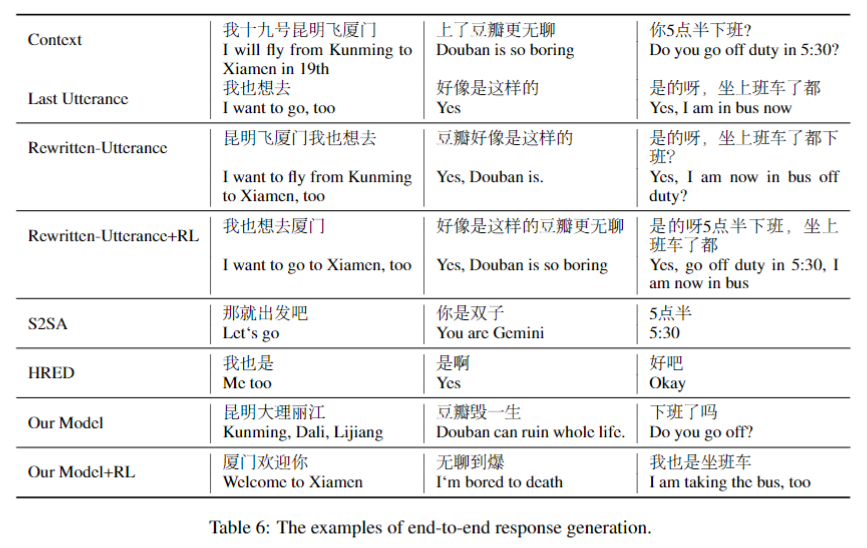# Unsupervised Context Rewriting for Open Domain Conversation

## Introduction• 有助于检索式chatbot的检索过程
• 有助于可解释和可控对话建模
• 重写后的结果可以让多轮问答变成单轮问答任务，单论问答的技术更加成熟。

## Model$$z_{t}=W_{f}^{T}\left[s_{t} ; \sum_{i=1}^{n q} \alpha_{q_{i}} h_{q_{i}} ; \sum_{i=1}^{n c} \alpha_{c_{i}} h_{c_{i}}\right]+b$$

\begin{aligned} \alpha_{i} &=\frac{\exp \left(e_{i}\right)}{\sum_{j=1}^{n} \exp \left(e_{j}\right)} \\ & e_{i}=h_{i} W_{a} s_{t} \end{aligned}

\begin{aligned} p\left(y_{t} | s_{t}, H_{Q}, H_{C}\right) &=p_{p r}\left(y_{t} | z_{t}\right) \cdot p_{m}\left(p r | z_{t}\right) \\ &+p_{c o}\left(y_{t} | z_{t}\right) \cdot p_{m}\left(c o | z_{t}\right) \end{aligned} \\ p_{m}\left(p r | z_{t}\right)=\frac{e^{\psi_{p r}\left(y_{t}, H_{Q}, H_{C}\right)}}{e^{\psi_{p r}\left(y_{t}, H_{Q}, H_{C}\right)}+e^{\psi_{c o}\left(y_{t}, H_{Q}, H_{C}\right)}}

Pre-training with Pseudo Data

$$\operatorname{PMI}\left(w_{c}, w_{r}\right)=-\log \frac{p_{c}\left(w_{c}\right)}{p\left(w_{c} | w_{r}\right)}$$

$w_{c}$ 是context word，$w_{r}$ 是response word。为了选择对回复来说最重要的词，作者也计算了$PMI(w_{c}, w_{q})$ （$w_{q}$是last utterance的词），最终的PMI分数为：
$$\operatorname{norm}\left(\operatorname{PMI}\left(w_{c}, q\right)\right)+\operatorname{norm}\left(\operatorname{PMI}\left(w_{c}, r\right)\right) \\ \operatorname{PMI}\left(w_{c}, q\right)=\sum_{w_{q} \in q} \operatorname{PMI}\left(w_{c}, w_{q}\right)$$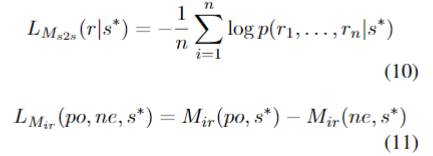Fine-Tuning with Reinforcement Learning

$$\nabla_{\theta} J(\theta)=E\left[R \cdot \nabla \log \left(P\left(y_{t} | x\right)\right)\right] \\ L_{c o m}=L_{r l}^{*}+\lambda L_{M L E}$$

• 回复生成：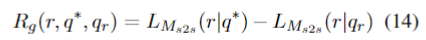• 回复选择：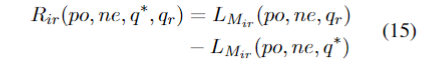## Experiments

Rewriting Quality Evaluation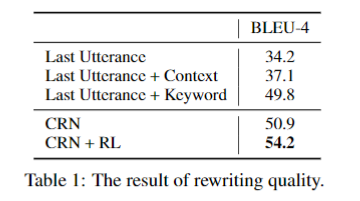Multi-turn Response Generation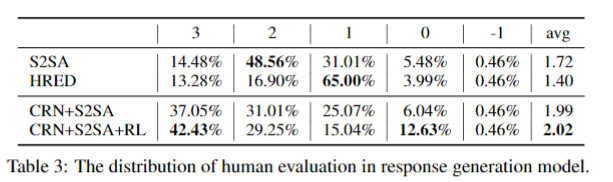Multi-turn Response Selection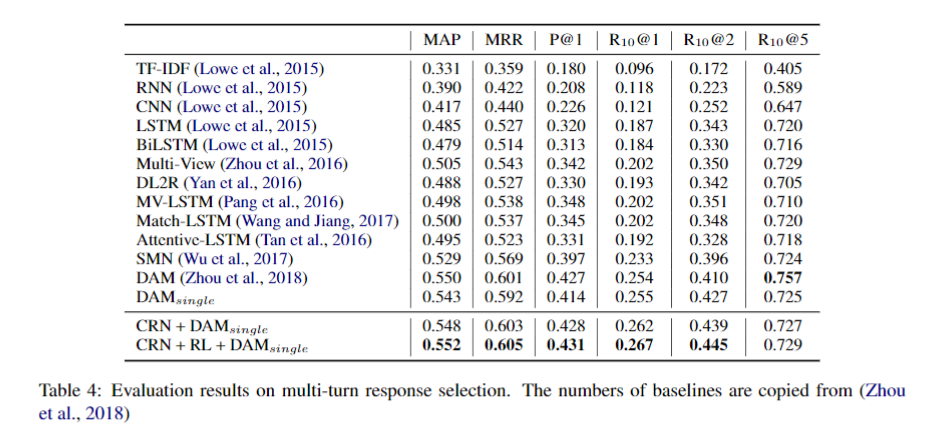End-to-End Multi-turn Response Selection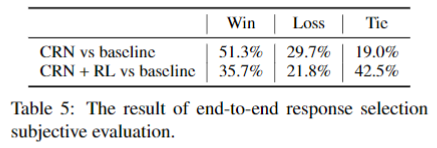Case Study